#### Vol. 48, No. 2, 1973

 Download this articleFor screen For printingRecent Issues Vol. 325: 1 Vol. 324: 1  2 Vol. 323: 1  2 Vol. 322: 1  2 Vol. 321: 1  2 Vol. 320: 1  2 Vol. 319: 1  2 Vol. 318: 1  2Online Archive Volume: Issue:The Journal Subscriptions Editorial Board Officers Contacts Submission Guidelines Submission Form Policies for Authors ISSN: 1945-5844 (e-only) ISSN: 0030-8730 (print) Special Issues Author Index To Appear Other MSP Journals
Matrix summability of a class of derived Fourier series

### Aribindi Satyanarayan Rao

Vol. 48 (1973), No. 2, 481–484
##### Abstract

Let f be L-integrable and periodic with period 2π, and let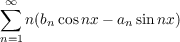(1.1)

be the derived Fourier series of the function f with partial sums sn(x). We write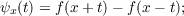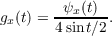In this paper, the following theorems are established.

Theorem 1. Let A = (amn) be a regular infinite matrix of real numbers. Then, for every x [π,π] for which gx(t) is of bounded variation on ,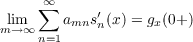(1.2)

if and only if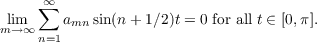(1.3)

Theorem 2. Let A = (amn) be an almost regular infinite matrix of real numbers. Then, for each x [π,π] for which gx(t) is of bounded variation on ,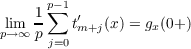uniformly in m if and only if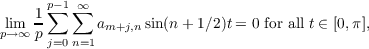uniformly in m, where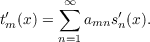Primary: 42A24
##### Milestones